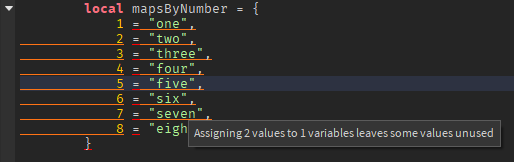# Error in a table

I have a table that gives me this error, how can i fix this?

``````		local mapsByNumber = {
1 = "one",
2 = "two",
3 = "three",
4 = "four",
5 = "five",
6 = "six",
7 = "seven",
8 = "eight"
}
``````

The error is: “Assigning 2 values to 1 variable leaves some values unused”

img:It looks like you want to make an array. Here is the correct way of doing it:

``````local mapsByNumber = {
 = "one",
 = "two",
 = "three",
 = "four",
 = "five",
 = "six",
 = "seven",
 = "eight"
}
``````
1 Like

This topic was automatically closed 14 days after the last reply. New replies are no longer allowed.